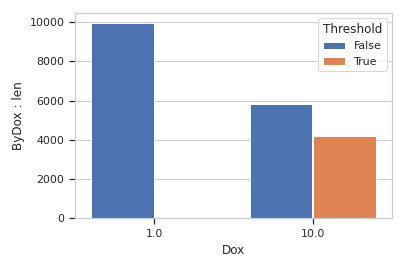# cytoflow.views.bar_chart¶

class cytoflow.views.bar_chart.BarChartView[source]

Plots a bar chart of some summary statistic

statistic

The name of the statistic to plot. Must be a key in the statistics attribute of the Experiment being plotted.

Type: (str, str)
error_statistic

The name of the statistic used to plot error bars. Must be a key in the statistics attribute of the Experiment being plotted.

Type: (str, str)
scale

The scale applied to the data before plotting it.

Type: {‘linear’, ‘log’, ‘logicle’}
variable

The condition that varies when plotting this statistic: used for the x axis of line plots, the bar groups in bar plots, etc.

Type: str
subset

An expression that specifies the subset of the statistic to plot.

Type: str
xfacet, yfacet

Set to one of the conditions in the Experiment, and a new row or column of subplots will be added for every unique value of that condition.

Type: String
huefacet

Set to one of the conditions in the in the Experiment, and a new color will be added to the plot for every unique value of that condition.

Type: String
huescale

How should the color scale for huefacet be scaled?

Type: {‘linear’, ‘log’, ‘logicle’}

Examples

Make a little data set.

>>> import cytoflow as flow
>>> import_op = flow.ImportOp()
>>> import_op.tubes = [flow.Tube(file = "Plate01/RFP_Well_A3.fcs",
...                              conditions = {'Dox' : 10.0}),
...                    flow.Tube(file = "Plate01/CFP_Well_A4.fcs",
...                              conditions = {'Dox' : 1.0})]
>>> import_op.conditions = {'Dox' : 'float'}
>>> ex = import_op.apply()


Add a threshold gate

>>> ex2 = flow.ThresholdOp(name = 'Threshold',
...                        channel = 'Y2-A',
...                        threshold = 2000).apply(ex)


>>> ex3 = flow.ChannelStatisticOp(name = "ByDox",
...                               channel = "Y2-A",
...                               by = ['Dox', 'Threshold'],
...                               function = len).apply(ex2)


Plot the bar chart

>>> flow.BarChartView(statistic = ("ByDox", "len"),
...                   variable = "Dox",
...                   huefacet = "Threshold").plot(ex3)enum_plots(experiment)[source]

Returns an iterator over the possible plots that this View can produce. The values returned can be passed to “plot”.

plot(experiment, plot_name=None, **kwargs)[source]

Plot a bar chart

Parameters: experiment (Experiment) – The Experiment to plot using this view. title (str) – Set the plot title xlabel, ylabel (str) – Set the X and Y axis labels huelabel (str) – Set the label for the hue facet (in the legend) legend (bool) – Plot a legend for the color or hue facet? Defaults to True. sharex, sharey (bool) – If there are multiple subplots, should they share axes? Defaults to True. row_order, col_order, hue_order (list) – Override the row/column/hue facet value order with the given list. If a value is not given in the ordering, it is not plotted. Defaults to a “natural ordering” of all the values. height (float) – The height of each row in inches. Default = 3.0 aspect (float) – The aspect ratio of each subplot. Default = 1.5 col_wrap (int) – If xfacet is set and yfacet is not set, you can “wrap” the subplots around so that they form a multi-row grid by setting col_wrap to the number of columns you want. sns_style ({“darkgrid”, “whitegrid”, “dark”, “white”, “ticks”}) – Which seaborn style to apply to the plot? Default is whitegrid. sns_context ({“paper”, “notebook”, “talk”, “poster”}) – Which seaborn context to use? Controls the scaling of plot elements such as tick labels and the legend. Default is talk. despine (Bool) – Remove the top and right axes from the plot? Default is True. orientation ({‘vertical’, ‘horizontal’}) lim ((float, float)) – Set the range of the plot’s axis. color (a matplotlib color) – Sets the colors of all the bars, even if there is a hue facet errwidth (scalar) – The width of the error bars, in points errcolor (a matplotlib color) – The color of the error bars capsize (scalar) – The size of the error bar caps, in points

Notes

Other kwargs are passed to matplotlib.axes.Axes.bar